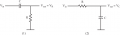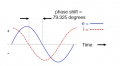# Rusty

#### ApacheKid

Joined Jan 12, 2015
1,269
I said I was rusty but I never realized just how much until yesterday evening.

I hooked up my scope to a trivial RC circuit, fed by a signal generator, and measured and displayed the voltages across the entire circuit and just the capacitor - Fig 2.

But the current was lagging the voltage not leading.Well this is what I saw:In my mind the peak point of e was followed by the peak point of i.

That is I was perceiving stuff to the left to be later than stuff on the right.

This is of course the exact opposite of reality, this is what can happen when one abandons a subject for too long !

#### ApacheKid

Joined Jan 12, 2015
1,269
I also have a question about Fig 2 (or Fig 1) - it is this:

At one stage I put one set of scope leads across the capacitor (so the lead ground was at ground) and the other across the resistor (so its ground lead was at the Vout point).

That caused all sort of weirdness because of course the scopes grounds are connected together.

How would one measure these two voltages independently and simultaneously given that the probes grounds are - in effect - connected together?

PS: Also on a digital scope is there a way to get just a slow scanning dot like we see on old analog scopes or is that just an artifact of old analog scopes?

I know there's no need to "scan" no concept of that really so perhaps it makes no sense to even ask such a dumb question...

#### nsaspook

Joined Aug 27, 2009
11,796
I also have a question about Fig 2 (or Fig 1) - it is this:

At one stage I put one set of scope leads across the capacitor (so the lead ground was at ground) and the other across the resistor (so its ground lead was at the Vout point).

That caused all sort of weirdness because of course the scopes grounds are connected together.

How would one measure these two voltages independently and simultaneously given that the probes grounds are - in effect - connected together?

PS: Also on a digital scope is there a way to get just a slow scanning dot like we see on old analog scopes or is that just an artifact of old analog scopes?

I know there's no need to "scan" no concept of that really so perhaps it makes no sense to even ask such a dumb question...
Usually you can set a DSO to X/Y or 'Roll' mode instead of normal scan.

Last edited:

#### MrChips

Joined Oct 2, 2009
29,251
Too many questions in one post. However, here goes.

1) A memory aid: ELI the ICE man.
E leads I in an inductor L.
I leads E in a capacitor C. (E = E.M.F = Volts)

2) In circuit (1), you are measuring the voltage across the resistor R. Hence V and I are in phase.

3) The scope is sweeping from left to right in time. Events on the left occur earlier than events on the right.
Hence i is leading e in the diagram. (Of course, everything repeats after 360°. Hence leading by 90° could be mistakenly construed as lagging by 270°)

4) With the scope grounded you can use both CHAN 1 and CHAN2 to measure the voltage at two nodes.
Then use the MATH function and use SUBTRACT to take the difference between the two voltages.
Also using the scope in X-Y mode as suggested is another way of showing phase relationship.

#### ApacheKid

Joined Jan 12, 2015
1,269
Too many questions in one post. However, here goes.

1) A memory aid: ELI the ICE man.
E leads I in an inductor L.
I leads E in a capacitor C. (E = E.M.F = Volts)

2) In circuit (1), you are measuring the voltage across the resistor R. Hence V and I are in phase.
I was actually measuring the voltage across the circuit and also across the capacitor, so I did see the phase difference.

3) The scope is sweeping from left to right in time. Events on the left occur earlier than events on the right.
Hence i is leading e in the diagram. (Of course, everything repeats after 360°. Hence leading by 90° could be mistakenly construed as lagging by 270°)

4) With the scope grounded you can use both CHAN 1 and CHAN2 to measure the voltage at two nodes.
Then use the MATH function and use SUBTRACT to take the difference between the two voltages.
Also using the scope in X-Y mode as suggested is another way of showing phase relationship.
Well I didn't want to see the difference, I wanted to simultaneously see the voltage across one component and the voltage across some other component, how do people actually do that when the two sets of probe share a common ground without effectively shorting the circuit at some point?

Do you mean that the difference signal is that signal? it is what we'd see if we were able to measure that component without this undesirable ground shorting it?

#### MrChips

Joined Oct 2, 2009
29,251
A voltage measurement is a measure of potential difference. You are always measuring the voltage at a node with reference to a reference node. In the case of an oscilloscope that is grounded the reference node is earth ground.

You can make two types of voltage measurements (which comes down to the same thing).

1) You can make a voltage measurement with respect to a common reference point (earth ground or circuit COMMON).

2) You can make a voltage measurement between two nodes A and B (where neither node is earth ground). This is commonly done in order to measure the voltage across a component such as a resistor or capacitor.

You can use a DMM to measure (1) and (2) since a DMM is floating.

You can use a multi-channel oscilloscope to measure (1) and (2) by using the MATH SUBTRACT function.
If you need to display two traces simultaneously for comparative time measurement, you can set one trace to be node B, for example, and a second trace to be node A - node B.

#### ApacheKid

Joined Jan 12, 2015
1,269
A voltage measurement is a measure of potential difference. You are always measuring the voltage at a node with reference to a reference node. In the case of an oscilloscope that is grounded the reference node is earth ground.

You can make two types of voltage measurements (which comes down to the same thing).

1) You can make a voltage measurement with respect to a common reference point (earth ground or circuit COMMON).

2) You can make a voltage measurement between two nodes A and B (where neither node is earth ground). This is commonly done in order to measure the voltage across a component such as a resistor or capacitor.

You can use a DMM to measure (1) and (2) since a DMM is floating.

You can use a multi-channel oscilloscope to measure (1) and (2) by using the MATH SUBTRACT function.
If you need to display two traces simultaneously for comparative time measurement, you can set one trace to be node B, for example, and a second trace to be node A - node B.
Thanks, I understand, so is it true that a scope's input can never be made to be "floating"? the concept does not exist for scopes?

#### MrChips

Joined Oct 2, 2009
29,251
Besides the MATH SUBTRACT function there are other ways to make a "high side" or "floating" measurement.

1) You can float the DUT (device under test) by using an isolation transformer if the DUT is mains operated.

2) You can float the oscilloscope by using an isolation transformer.

3) You can use a hand-held battery operated oscilloscope. Inexpensive "hobby" scopes advertised on the internet are commonly powered by a AC wall adapter. If these are galvanically isolated from AC mains then the adapter and oscilloscope are floating.

#### ApacheKid

Joined Jan 12, 2015
1,269
Besides the MATH SUBTRACT function there are other ways to make a "high side" or "floating" measurement.

1) You can float the DUT (device under test) by using an isolation transformer if the DUT is mains operated.

2) You can float the oscilloscope by using an isolation transformer.

3) You can use a hand-held battery operated oscilloscope. Inexpensive "hobby" scopes advertised on the internet are commonly powered by a AC wall adapter. If these are galvanically isolated from AC mains then the adapter and oscilloscope are floating.
OK I understand that part I think, but even with that there seems to be no way to float the ground sides of the input channels, no matter what one does all the scope's input channels always have their respective grounds connected together - is that true?

#### MrChips

Joined Oct 2, 2009
29,251
OK I understand that part I think, but even with that there seems to be no way to float the ground sides of the input channels, no matter what one does all the scope's input channels always have their respective grounds connected together - is that true?
Ah yes, you are correct. I forgot about that part.